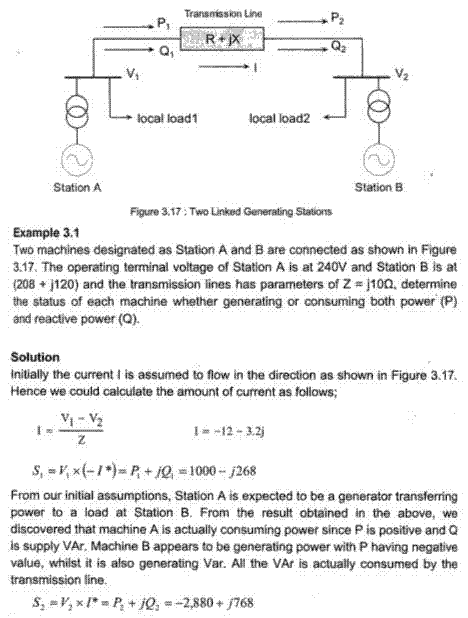# Is the apparent power calculation in this text WRONG?

Status
Not open for further replies.

#### powersysapparent power calculation

The attached example was scanned from a textbook. I tried to calculate S1 but the answer I got was different from the one shown in text. Could someone please help me to verify whether the solution provided in the text is CORRECT or WRONG?

My calculation:

I
= (V1 - V2) / Z
= (240 - 208 - j120) / j10
= -12-j3.2

S1
= V1 x (-I*)
= 240 x (12-j3.2)
= 2880-j768

Question: Why a negative '-' sign in front of I* is required for the calculation of S1? Pls advise.

S2
= V2 x (I*)
= (208+j120) X (-12+j3.2)
= -2880-j774.4#### smxx

##### Full Member level 4hi
I think that it is wrong

#### powersyssmxx said:
I think that it is wrong
Err... our answers (S1 and S2) are different. Why?
Do you think the "I" used in your calculation for S2 should be "conjugated"? Thanks.

Status
Not open for further replies.